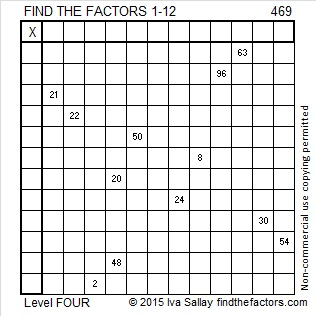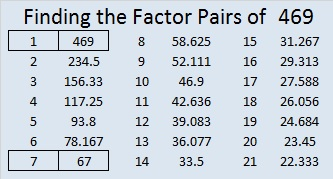# 469 and Level 4

469 is the short leg in the Pythagorean triple 469-1608-1675. What is the greatest common factor of those three numbers? Hint: Don’t let the larger numbers scare you; the greatest common factor is a factor of 469, the smallest of those three numbers, and its factors are listed below the puzzle.Print the puzzles or type the solution on this excel file:  12 Factors 2015-04-20

—————————————————————————————————

• 469 is a composite number.
• Prime factorization: 469 = 7 x 67
• The exponents in the prime factorization are 1 and 1. Adding one to each and multiplying we get (1 + 1)(1 + 1) = 2 x 2 = 4. Therefore 469 has exactly 4 factors.
• Factors of 469: 1, 7, 67, 469
• Factor pairs: 469 = 1 x 469 or 7 x 67
• 469 has no square factors that allow its square root to be simplified. √469 ≈ 21.6564—————————————————————————————————